AP State Board Syllabus AP SSC 10th Class Maths Textbook Solutions Chapter 8 Similar Triangles Ex 8.4 Textbook Questions and Answers.

## AP State Syllabus SSC 10th Class Maths Solutions 8th Lesson Similar Triangles Exercise 8.4

### 10th Class Maths 8th Lesson Similar Triangles Ex 8.4 Textbook Questions and Answers

Question 1.
Prove that the sum of the squares of the sides of a rhombus is equal to the sum of the squares of its diagonals.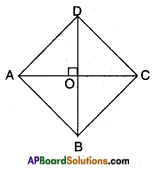Given : □ ABCD is a rhombus.
Let its diagonals AC and BD bisect each other at ‘O’.
We know that “the diagonals in a rhombus are perpendicular to each other”.
In △AOD; AD2 = OA2 + OD2 ………. (1)
[Pythagoras theorem]
In △COD; CD2 = OC2 + OD2 ………. (2)
[Pythagoras theorem]
In △AOB; AB2 = OA2 + OB2 ………. (3)
[Pythagoras theorem]
In △BOC; BC2 = OB2 + OC2 ………. (4)
[Pythagoras theorem]
Adding the above equations we get AD2 + CD2 + AB2 + BC2 = 2 (OA2 + OB2 + OC2 + OD2)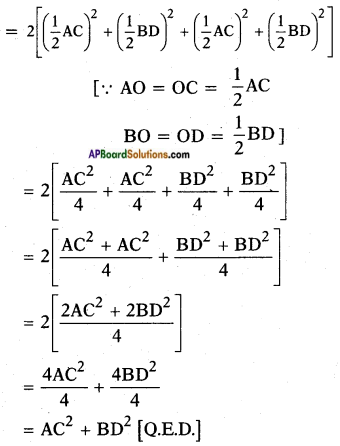Question 2.
ABC is a right triangle right angled at B. Let D and E be any points on AB and BC respectively. Prove that AE2 + CD2 = AC2 + DE2.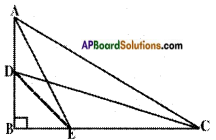Given: In △ABC; ∠B = 90°
D and E are points on AB and BC.
R.T.P.: AE2 + CD2 = AC2 + DE2
Proof: In △BCD, △BCD is a right triangle right angled at B.
∴ BD2 + BC2 = CD2 ……… (1)
[∵ Pythagoras theorem states that hypotenuse2 = side2 + side2]
In △ABE; ∠B = 90°
Adding (1) and (2), we get
BD2 + BC2 + AB2 + BE2 – CD2 + AE2
(BD2 + BE2) + (AB2 + BC2) = CD2 + AE2
DE2 + AC2 – CD2 + AE2 [Q.E.D.]
[∵ (i) In △DBE, ∠B = 90° and DE2 = BD2 + BE2
(ii) In △ABC, ∠B = 90° and AB2 + BC2]

Question 3.
Prove that three times the square of any side of an equilateral triangle is equal to four times the square of the altitude.
Given: △ABC, an equilateral triangle;
AD – altitude and the side is a units, altitude h units.
R.T.P: 3a2 = 4h2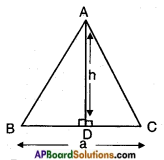Proof: In △ABD, △ACD
∠B = ∠C [∵ 60°]
∴ ∠BAD = ∠DAC [∵ Angle sum property]
Also, BA = CA
∴ △ABD s △ACD (by SAS congruence condition)
Hence, BD = CD = $$\frac{1}{2}$$BC = $$\frac{a}{2}$$ [∵ c.p.c.t]
Now in △ABD, AB2 = AD2 + BD2
[∵ Pythagoras theorem]
a2 = h2 + $$\left(\frac{a}{2}\right)^{2}$$
a2 = h2 + $$\frac{a^{2}}{4}$$
h2 = $$\frac{4 a^{2}-a^{2}}{4}$$
∴ h2 = $$\frac{3 a^{2}}{4}$$
⇒ 4h2 = 3a2 (Q.E.D)Question 4.
PQR is a triangle right angled at P and M is a point on QR such that PM ⊥ QR. Show that PM2 = QM . MR.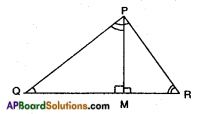Given: In △PQR, ∠P = 90° and PM ⊥ QR.
R.T.P : PM2 = QM . MR
Proof: In △PQR; △MPR
∠P = ∠M [each 90°]
∠R = ∠R [common]
∴ △PQR ~ △MPR ……… (1)
[A.A. similarity]
In △PQR and △MQP,
∠P = ∠M (each 90°)
∠Q = ∠Q (common)
∴ △PQR ~ △MQP ……… (2)
[A.A. similarity]
From (1) and (2),
△PQR ~ △MPR ~ △MQP [transitive property]
∴ △MPR ~ △MQP
$$\frac{MP}{MQ}$$ = $$\frac{PR}{QP}$$ = $$\frac{MR}{MP}$$
[Ratio of corresponding sides of similar triangles are equal]
$$\frac{PM}{QM}$$ = $$\frac{MR}{PM}$$
PM . PM = MR . QM
PM2 = QM . MR [Q.E.D]

Question 5.
ABD is a triangle right angled at A and AC ⊥ BD.
Show that (i) AB2 = BC BD
(iii) AC2 = BC DC.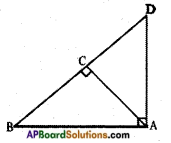Given: In △ABD; ∠A = 90° AC ⊥ BD
R.T.P.:
i) AB2 = BC . BD
Proof: In △ABD and △CAB,
∠B = ∠B [common]
∴ △ABD ~ △CBA
[by A.A. similarity condition]
Hence, $$\frac{AB}{BC}$$ = $$\frac{BD}{AB}$$ = $$\frac{AD}{AC}$$
[∵ Ratios of corresponding sides of similar triangles are equal]
$$\frac{AB}{BD}$$ = $$\frac{BC}{AB}$$
⇒ AB . AB = BC . BD
∴ AB2 = BC . BDii) AD2 = BD . CD
∠D = ∠D (common)
∴ △ABD ~ △CAD [A.A similarity]
Hence, $$\frac{AB}{AC}$$ = $$\frac{BD}{AD}$$ = $$\frac{AD}{CD}$$
⇒ $$\frac{BD}{AD}$$ = $$\frac{AD}{CD}$$
AD2 = BD . CD [Q.E.D]

iii) AC2 = BC . DC
Proof: From (i) and (ii)
△ACB ~ △DCA
[∵ △BAD ~ △BCA ~ △ACD
Hence, $$\frac{AC}{DC}$$ = $$\frac{BC}{AC}$$ = $$\frac{AB}{AD}$$
$$\frac{AC}{DC}$$ = $$\frac{BC}{AC}$$
AC . AC = BC . DC
AC2 = BC . DC [Q.E.D]

Question 6.
ABC is an isosceles triangle right angled at C. Prove that AB2 = 2AC2.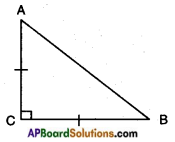Given: In △ABC; ∠C = 90°; AC = BC.
R.T.P.: AB2 = 2AC2
Proof: In △ACB; ∠C = 90°
Hence, AC2 + BC2 = AB2
[Square of the hypotenuse is equal to sum of the squares of the other two sides – Pythagoras theorem]
⇒ AC2 + AC2 = AB2 [∵ AC = BC given]
⇒ AB2 = 2AC2 [Q.E.D.]Question 7.
‘O’ is any point in the interior of a triangle ABC.
OD ⊥ BC, OE ⊥ AC and OF ⊥ AB, show that
i) OA2 + OB2 + OC2 – OD2 – OE2 – OF2 = AF2 + BD2 + CE2
ii) AF2 + BD2 + CE2 = AE2 + CD2 + BF2.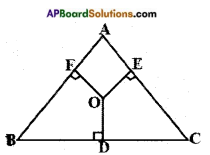Given: △ABC; O’ is an interior point of △ABC.
OD ⊥ BC, OE ⊥ AC, OF ⊥ AB.
R.T.P.:
i) OA2 + OB2 + OC2 – OD2 – OE2 – OF2 = AF2 + BD2 + CE2
Proof: In OAF, OA2 = AF2 + OF2 [Pythagoras theorem]
⇒ OA2 – OF2 = AF2 …….. (1)
In △OBD,
OB2 = BD2 + OD2
⇒ OB2 – OD2 = BD2 …….. (2)
In △OCE, OC2 = CE2 + OE2
OC2 – OE2 = CE2 ……… (3)
Adding (1), (2) and (3) we get,
OA2 – OF2 + OB2 – OD2 + OC2 – OE2 = AF2 + BD2 + CE2
OA2 + OB2 + OC2 – OD2 – OE2 – OF2 = AF2 + BD2 + CE2 ……… (4)

ii) AF2 + BD2 + CE2 = AE2 + CD2 + BF2
In △OAE,
OA2 = AE2 + OF2 ……… (1)
⇒ OA2 – OE2 = AE2
In △OBF, OB2 = BF2 + OF2
OB2 – OF2 = BF2 ……… (2)
In △OCD, OC2 = OD2 + CD2
OC2 – OD2 = CD2 ……… (3)
Adding (1), (2) and (3) we get
OA2 – OE2 + OB2 – OF2 + OC2 – OD2 = AE2 + BF2 + CD2
⇒ OA2 + OB2 + OC2 – OD2 – OE2 – OF2 = AE2 + CD2 + BF2
⇒ AF2 + BD2 + CE2 = AE2 + CD2 + BF2 [From problem (i)]

Question 8.
A wire attached to vertical pole of height 18 m is 24 m long and has a stake attached to the other end. How far from the base of the pole should the stake be driven so that the wire will be taut?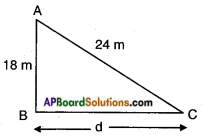Height of the pole AB = 18 m.
Length of the wire AC = 24 m.
Distance beween the pole and the stake be ‘d’ meters.
By Pythagoras theorem,
Hypotenuse2 = side2 + side2
242 = 182 + d2
d2 = 242 – 182 = 576 – 324 = 252
= $$\sqrt{36 \times 7}$$
∴ d = 6√7 m.Question 9.
Two poles of heights 6 m and 11m stand on a plane ground. If the distance between the feet of the poles is 12 m, find the distance between their tops.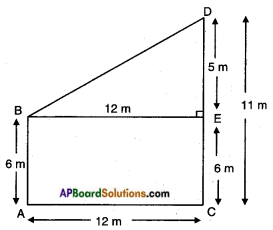Let the height of the first pole AB = 6 m.
Let the height of the second pole CD = 11 m.
Distance between the poles AC = 12 m.
From the figure □ ACEB is a rectangle.
∴ AB = CE = 6 m
ED = CD – CE = 11 – 6 = 5 m
Now in △BED; ∠E = 90°; DE = 5 m; BE = 12 m
BD2 = BE2 + DE2
[hypotenuse2 = side2 + side2 – Pythagoras theorem]
= 122 + 52
= 144 + 25
BD2 = 169
BD = √l69 = 13m
∴ Distance between the tops of the poles = 13 m.

Question 10.
In an equilateral triangle ABC, D is a point on side BC such that BD = $$\frac{1}{3}$$ BC. Prove that 9AD2 = 7AB2.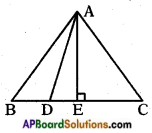In △ABE, ∠E = 90°
⇒ $$\overline{\mathrm{AB}}$$ is hypotenuse.
∴ AB2 = AE2 + BE2
AE2 = AB2 – BE2
⇒ AE2 = AB2 – $$\left(\frac{BC}{2}\right)^{2}$$
= AE2 = AB2 – $$\left(\frac{AB}{2}\right)^{2}$$ (∵ AB = BC)
⇒ AE2 = $$\frac{3}{4}$$AB2 ……… (1)
⇒ $$\overline{\mathrm{AD}}$$ is hypotenuse.
⇒ AD2 = AE2 + DE2
⇒ AE2 = AD2 + DE2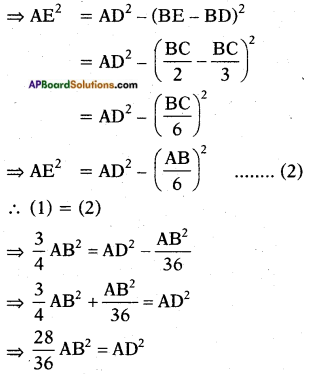⇒ 28 AB2 = 36 AD2
⇒ 7 AB2 = 9 AD2
Hence proved.Question 11.
In the given figure, ABC is a triangle right angled at B. D and E are points on BC trisect it. Prove that 8 AE2 = 3 AC2 + 5 AD2.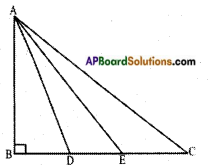In △ABC, ∠B=90°
⇒ $$\overline{\mathrm{AD}}$$ is hypotenuse.
AC2 = AB2 + BC2
3AC2 = 3AB2 + 3BC2 …….. (1)
In △ABD, ∠B = 90°
∴ AD2 = AB2 + BD2 = AB2 + $$\left(\frac{BC}{3}\right)^{2}$$
⇒ AD2 = AB2 + $$\frac{\mathrm{BC}^{2}}{9}$$
⇒ 5 AD2 = 5 AB2 + $$\frac{5 \mathrm{BC}^{2}}{9}$$ …….. (2)
(1) + (2)
3 AC2 + 5 AD2 = 3 AB2 + 3 BC2 + 5 AB2 + $$\frac{5}{9} \mathrm{BC}^{2}$$
= 8AB2 + $$\frac{32}{9} \mathrm{BC}^{2}$$ ……… (3)
Now in △ABE, ∠B = 90°
⇒ $$\overline{\mathrm{AE}}$$ is hypotenuse.
⇒ AE2 = AB2 + BE2 = AB2 + $$\left(\frac{2}{3} BC\right)^{2}$$
= AB2 + $$\frac{4}{9} \mathrm{BC}^{2}$$
⇒ AE2 = 8AB2 + $$\frac{32}{9} \mathrm{BC}^{2}$$ ……… (4)
∴ RHS of (3) and (4) are equal.
∴ LHS of (3) and (4) are equal.
∴ 8 AE2 = 3 AC2 + 5 AD2.
Hence proved.

Question 12.
ABC is an isosceles triangle right angled at B. Equilateral triangles ACD and ABE are constructed on sides AC and AB. Find the ratio between the areas of △ABE and △ACD.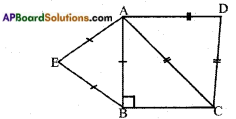$$\frac{\Delta \mathrm{ABE}}{\Delta \mathrm{ACD}}$$ = $$\frac{\mathrm{AB}^{2}}{\mathrm{AC}^{2}}$$
= $$\frac{a^{2}}{2 a^{2}}$$ = $$\frac{1}{2}$$